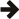#Boost C++ Libraries

...one of the most highly regarded and expertly designed C++ library projects in the world.## Chapter 1. Boost.Functional/OverloadedFunction 1.0.0

Distributed under the Boost Software License, Version 1.0 (see accompanying file LICENSE_1_0.txt or copy at http://www.boost.org/LICENSE_1_0.txt)

This library allows to overload different functions into a single function object.

## Introduction

Consider the following functions which have distinct signatures:

```const std::string& identity_s(const std::string& x) // Function (as pointer).
{ return x; }

int identity_i_impl(int x) { return x; }
int (&identity_i)(int) = identity_i_impl; // Function reference.

double identity_d_impl(double x) { return x; }
boost::function<double (double)> identity_d = identity_d_impl; // Functor.
```

Instead of calling them using their separate names (here `BOOST_TEST` is equivalent to `assert`): 

```BOOST_TEST(identity_s("abc") == "abc");
BOOST_TEST(identity_i(123) == 123);
BOOST_TEST(identity_d(1.23) == 1.23);
```

It is possible to use this library to create a single overloaded function object (or functor) named `identity` that aggregates together the calls to the specific functions (see also `functor.cpp` and `identity.hpp`):

```boost::overloaded_function<
const std::string& (const std::string&)
, int (int)
, double (double)
> identity(identity_s, identity_i, identity_d);

// All calls via single `identity` function.
BOOST_TEST(identity("abc") == "abc");
BOOST_TEST(identity(123) == 123);
BOOST_TEST(identity(1.23) == 1.23);
```

Note how the functions are called via a single overloaded function object `identity` instead of using their different names `identity_s`, `identity_i`, and `identity_d`.

 In most of the examples presented in this documentation, the Boost.Core/LightweightTest (`boost/core/lightweight_test.hpp`) macro `BOOST_TEST` is used to check correctness conditions (conceptually similar to `assert`). A failure of the checked condition does not abort the execution of the program, it will instead make `boost::report_errors` return a non-zero program exit code. Using Boost.Core/LightweightTest allows to add the examples to the library regression tests so to make sure that they always compile and run correctly.

 Last revised: April 13, 2021 at 16:29:22 GMT